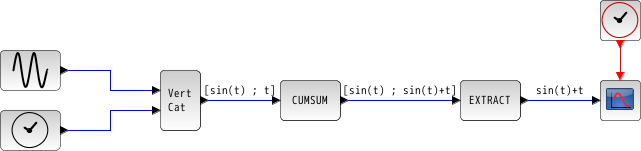Change language to:
English - Français - Português - Русский

See the recommended documentation of this function

Scilabヘルプ >> Xcos > palettes > Matrix operation palette > CUMSUM

# CUMSUM

Cumulative Sum

### Block Screenshot### Description

The CUMSUM block cumulatively sums the element of an input MxN matrix U along either the rows, the columns or the first non singleton dimension. The size of the output is also MxN.

When the Sum along parameter is set to:

• 1: the block progressively sums across the elements of each row.

• 2: the block progressively sums across the elements of each column.

• 0: the block progressively sums across the first non singleton dimension.

The equivalent function in Scilab is cumsum.

### Dialog box• Datatype(1=real double 2=Complex)

It indicates the type of the output. It support only the two types double (1) and complex (2). If we input another entry in this label Scicos will print the message "Datatype is not supported".

Properties : Type 'vec' of size 1.

• Sum along (0=the first non singleton dimension 1=Rows 2=Columns)

Indicate whether to cumulatively sum across the rows, the columns or the first non singleton dimension.

Properties : Type 'vec' of size 1.

### Example

```A = [1 2 3 ; 4 5 6 ; 7 8 9]
If the sum is along the row the result will be:
B = [1 2 3 ; 5 7 9 ; 12 15 18]```

### Default properties

• always active: no

• direct-feedthrough: yes

• zero-crossing: no

• mode: no

• regular inputs:

- port 1: size [-1,-2] / type 1

• regular outputs:

- port 1: size [-1,-2] / type 1

• number/sizes of activation inputs: 0

• number/sizes of activation outputs: 0

• continuous-time state: no

• discrete-time state: no

• object discrete-time state: no

• name of computational function: cumsum_m

### Interfacing function

• SCI/modules/scicos_blocks/macros/MatrixOp/CUMSUM.sci

### Computational function

• SCI/modules/scicos_blocks/src/c/cumsum_m.c

• SCI/modules/scicos_blocks/src/c/cumsum_r.c

• SCI/modules/scicos_blocks/src/c/cumsum_c.c

• SCI/modules/scicos_blocks/src/c/cumsumz_m.c

• SCI/modules/scicos_blocks/src/c/cumsumz_r.c

• SCI/modules/scicos_blocks/src/c/cumsumz_c.c

### Cumulative Sum example